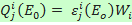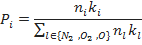Effective Energetic Costs of Excitation of Electronic States of N2, O2, and O by Electrons

Introduction

The effective energetic costs are numerical quantities defined to simplify estimation of excitation rates of electronic states of the Earth’s atmospheric gases by electron impact. In was shown  that for energies >1 keV the energetic costs are typically independent on initial electron energy and on the altitude in atmosphere. This approach is mainly valid for aeronomic applications (aurora). The constant energetic costs are usually enough for typical applications to electron-excited aurora, but this approach cannot be straightforwardly applied, for example, to secondary electrons produced by other impact particles, and in case of some exceptions.  The exceptions are: i) dependence on altitude for states with low (<7 eV) thresholds; and ii) strong dependence on initial electron energy in region of thresholds (<50 eV). Considerable density of thermal electrons is typical for ionospheric altitudes, so the electron-electron interaction can also modify the energetic costs at small energies.

So, in common case the energetic cost εji of excitation of a state j of a gas i by electrons of energy Eo should depends on gas composition, on initial energy of electrons Eo, and on possible admixture of thermal electrons. The results presented on this web-site illustrate which of these dependences are important and where.

How it was obtained

The energetic costs have been calculated numerically. The numerical model used is the Monte-Carlo code which simulates degradation of electron energy within collision-by-collision scheme down to the lowest threshold energy. All generations of secondary electrons are also taken into account sequentially. The local degradation was assumed and the gas composition can be an arbitrary mixture of 3 gases: molecular nitrogen, molecular oxygen, and atomic oxygen. For illustration we have presented results for homogeneous gas and mixtures which are typical at auroral altitudes 100 km, 120 km and 200 km.

The partial cross sections used in the numerical model are mainly the same as in [1, 2]. The only improvements based on [3-8] are: i) more careful shapes for vibrational states (v=1,2,3,4) of molecular nitrogen and molecular oxygen at small energies; ii) the rotation states (r=1,2,3,4) of molecular nitrogen are added.

The electron-electron interaction is included to the simulation by effective loss function obtained by :

Lee [eV cm-2] = 3.1* 10-12/E [eV], here E is current energy of the non-thermal electron.

Statistical discrepancy of all calculated valued are less than 0.1%, therefore the main reason of possible errors is the partial collisional cross sections which are typically known with discrepancy >30%.

How to apply

The excitation rate of given state j of gas i can be calculated as:,

Here Wi is the energy lost by electron flux in collisions with gas i. For mixture of gases (air) the energy can be calculated as a fraction of total energy flux of electrons:

Wi = Eo Ne Pi , where Ne is number flux in the energetic electron beam, andHere ni is density of gas i, the constants are kN2=1, kO2=0.7, kO=0.4.

More details see in  and [2, chapter 7].

References

1. Sergienko T.I., Ivanov V.E. A new approach to calculate the excitation of atmospheric gases by auroral electron impact, Ann. Geophys.  V.11,  P.717, 1993.

2. Ivanov V.E., Kozelov B.V., Transport of electron and proton-hydrogen atom fluxes in the Earth atmosphere. Published by KSC RAS, Apatity, 2001 (book in Russian, pdf-file).

3. Allan M., Excitation of vibrational levels up to v=17 in N2 by electron in the 0-5 eV region, J. Phys. B: At. Mol. Phys. 18 (1985) 4511-4517.

4. Vicic M., Poparic G., Belic D.S., Large vibrational excitation of N2 by low-energy electrons, J. Phys. B: At. Mol. Opt. Phys. 29 (1996) 1273–1281.

5. Brennan M. J., Alle D. T., Euripidest P., Buckman S. J.  and M. J. Brungert, Elastic electron scattering and rovibrational excitation of N2 at low incident energies, J. Phys. B: At. Mol. Opt. Phys. 25 (1992) 2669-2682.

6. Gote M. and H. Ehrhardt, Rotational excitation of diatomic molecules at intermediate energies: absolute differential state-to-state transition cross sections for electron scattering from N2, Cl2, CO and HCl, J. Phys. B: At. Mol. Opt. Phys. 28 (1995) 3957-3986.

7. Kutz H.and Meyer H-D, Rotational excitation of N2 and Cl2 molecules by electron impact in the energy range 0.01-1000 eV: Investigation of excitation mechanisms, Physical Rev. A, V.51 (5), 3819-3830, 1995.

8. Campbell L., M.J. Brunger, D.C. Cartwright, P.J.O. Teubner, Production of vibrationally excited N2 by electron impact, Planet. Space Sci., 52 815-822, 2004.

9. Schunk R.W., Hays P.B. Photoelectron energy losses to thermal electrons, Planet. Space Sci. (1971) - V.19. - P.113-117.

Version 2013-08-03
(c) Boris Kozelov, PGI KSC RAS, E-mail: boris.kozelov@gmail.com